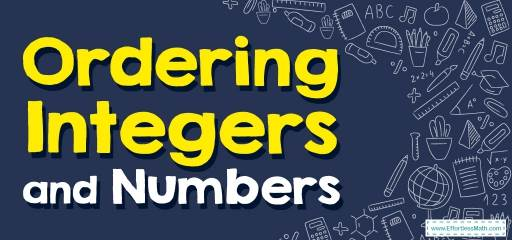# How to Order Integers and Numbers? (+FREE Worksheet!)

We can use a number line for ordering integers and numbers. We teach you how to order integers and numbers in a few simple steps. So join us!Any number that can be displayed without a fraction is called an integer. For example, the numbers $$−10, −5, 0, 1, 2$$ are integers because we can specify them without having to display a regular fraction.

It can be said that integers consist of three categories:

1. Positive integers
2. Zero
3. Negative integers

We can use a number line for ordering numbers and integers. Learn how to order numbers in a few simple steps.

## Step by step guide to ordering integers and numbers

When using a number line, the more we go to the positive (right), the bigger the numbers become, and the more we go to the negative (left), the smaller the numbers become.

When comparing two numbers, think about their position on the number line. If one number is on the right side of another number, it is a bigger number. For example, $$- \ 3$$ is bigger than $$- \ 5$$ because it is on the right side of $$- \ 5$$ on number line.

To order integers and numbers:

• Step 1: For the first step, look at the smallest numbers, which are the negative numbers that are farthest from zero, and arrange them. The bigger the negative number, the smaller the value.
• Step 2: Zero is bigger than all negative numbers, so in ordering numbers, it ranks higher than negative.
• Step 3: We said that the more numbers move to the right, the bigger they are, so the positive numbers are after zero. The bigger the positive number, the bigger the value.

### Ordering Integers and Numbers – Example 1:

Order this set of integers from least to greatest. $$- 4, – 1, – 5, 4, 2, 7$$

Solution:

The smallest number is $$- \ 5$$ and the largest number is $$7$$.
Now compare the integers and order them from least to greatest:
$$- 5 \ < \ – 4 \ < \ -1 \ < \ 2 \ < \ 4 \ < \ 7$$

### Ordering Integers and Numbers – Example 2:

Order this set of integers from greatest to least. $$3, – 2, -1, 6, -9, 8$$

Solution:

The largest number is $$8$$ and the smallest number is $$- \ 9$$.
Now compare the integers and order them from greatest to least:
$$8 \ > \ 6 \ > \ 3 \ > \ – \ 1 \ > \ – \ 2 \ > \ – \ 9$$

### Ordering Integers and Numbers – Example 3:

Order this set of integers from least to greatest. $$-2,1,-5,-1,2,4$$

Solution:

The smallest number is $$-5$$ and the largest number is $$4$$.
Now compare the integers and order them from greatest to least:
$$-5<-2<-1<1<2<4$$

### Ordering Integers and Numbers – Example 4:

Order each set of integers from greatest to least. $$10,-6,-2,5,-8,4$$

Solution:

The largest number is $$10$$ and the smallest number is $$-8$$.
Now compare the integers and order them from least to greatest:
$$10>5>4>-2>-6>-8$$

## Exercises for Ordering Integers and Numbers

### Order each set of integers from least to greatest.

• $$\color{blue}{ – 15, – 19, 20, – 4, 1 }$$
• $$\color{blue}{ 6, – 5, 4, – 3, 2}$$
• $$\color{blue}{ 15, – 42, 19, 0, – 22 }$$

### Order each set of integers from greatest to least.

• $$\color{blue}{ – 2, 5, – 3, 6, – 4 }$$
• $$\color{blue}{ – 37, 7, – 17, 27, 47 }$$
• $$\color{blue}{ 32, – 27, 19, – 17, 15 }$$

• $$\color{blue}{ – 19, – 15, – 4, 1, 20 }$$
• $$\color{blue}{ – 5, – 3, 2, 4, 6 }$$
• $$\color{blue}{ – 42, – 22, 0, 15, 19}$$
• $$\color{blue}{6, 5, – 2, – 3, – 4}$$
• $$\color{blue}{47, 27, 7, – 17, – 37}$$
• $$\color{blue}{32, 19, 15, – 17, – 27}$$

### What people say about "How to Order Integers and Numbers? (+FREE Worksheet!) - Effortless Math: We Help Students Learn to LOVE Mathematics"?

No one replied yet.

X
30% OFF

Limited time only!

Save Over 30%

SAVE $5 It was$16.99 now it is \$11.99